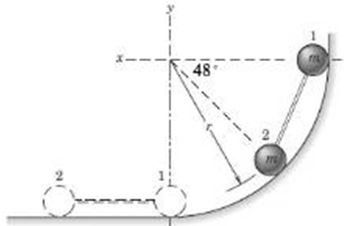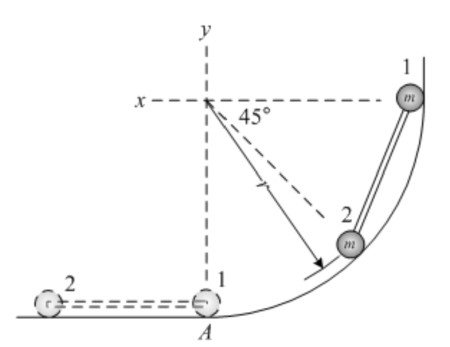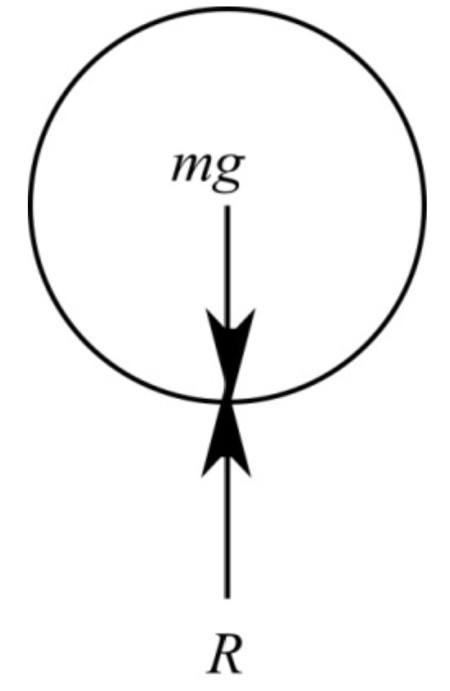# The two small spheres, each of mass m = 1.5 kg, are rigidly connected by a rod of negligible mass...

## Question:

The two small spheres, each of mass m = 1.5 kg, are rigidly connected by a rod of negligible mass and are released from rest in the position shown and slide down the smooth circular guide in the vertical plane. The distance r = 0.77 m. Determine their common velocity v as they reach the horizontal dashed position. Also find the force R between sphere 1 and the supporting surface an instant before the sphere reaches the bottom position A.## Energy conservation

Conservation of energy is the principle that energy is not created nor destroyed; it only moves from one place to another - from one type of energy to another. There are many types of energy. For example, a fan turns electrical energy into mechanical energy.

Free body diagram of the systemThe system is initially at rest

Initial kinetic energy {eq}T_i=0 {/eq}

Initial potential energy

{eq}V_i=\sum m_igr_i\\ =m_1gr_1+m_2gr_2....(1) r_1=r\\ r_2=r-rsin45^\circ\\ =r(1-sin48^\circ)\\ V_i=mgr+mgr(1-sin48^\circ) =1.256mgr {/eq}

The final kinetic energy of the system

{eq}T_f=\dfrac{1}{2}m_1v_1^2+\dfrac{1}{2}m_2v_2^2.....(2)\\ T_f=\dfrac{1}{2}mv^2+\dfrac{1}{2}mv^2\\ =mv^2 {/eq}

Calculate the final potential energy of the system

The system comes to ground finally

{eq}V_f=0 {/eq}

Apply conservation of energy principle as shown

{eq}T_i+V_i=T_f+V_f\\ 0+1.256mgr=mv^2+0\\ v^2=1.256gr\\ v=\sqrt{1.256gr}\\ v=1.120(gr)^{\frac{1}{2}} {/eq}

Therefore the final velocity of the system of particles is {eq}v=1.120(gr)^{\frac{1}{2}} {/eq}

Free body diagram of sphere 1 as shownWrite the equilibrium equation

{eq}R-mg=\frac{mv^2}{R}\\ R-mg=\dfrac{m(1.136(gr)^{\frac{1}{2}})^2}{r}\\ R=mg+1.256mg\\ R=2.256mg {/eq}

Therefore reaction force is 2.256mg